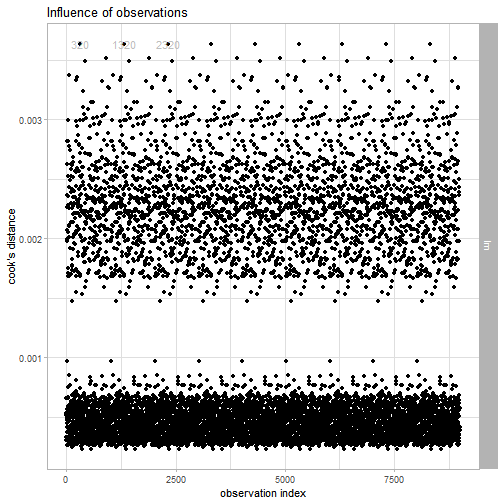# Regression use case - apartments data

To illustrate applications of auditor to regression problems we will use an artificial dataset apartments available in the DALEX package. Our goal is to predict the price per square meter of an apartment based on selected features such as construction year, surface, floor, number of rooms, district. It should be noted that four of these variables are continuous while the fifth one is a categorical one. Prices are given in Euro.

``````library(DALEX)
data("apartments")
``````
``````##   m2.price construction.year surface floor no.rooms    district
## 1     5897              1953      25     3        1 Srodmiescie
## 2     1818              1992     143     9        5     Bielany
## 3     3643              1937      56     1        2       Praga
## 4     3517              1995      93     7        3      Ochota
## 5     3013              1992     144     6        5     Mokotow
## 6     5795              1926      61     6        2 Srodmiescie
``````

# Models

## Linear model

``````lm_model <- lm(m2.price ~ construction.year + surface + floor + no.rooms + district, data = apartments)
``````

## Random forest

``````library("randomForest")
set.seed(59)
rf_model <- randomForest(m2.price ~ construction.year + surface + floor +  no.rooms + district, data = apartments)
``````

# Preparation for error analysis

The beginning of each analysis is creation of a `modelAudit` object. It’s an object that can be used to audit a model.

``````library("auditor")

lm_audit <- audit(lm_model, label = "lm", data = apartmentsTest, y = apartmentsTest\$m2.price)
rf_audit <- audit(rf_model, label = "rf", data = apartmentsTest, y = apartmentsTest\$m2.price)
``````

# Audit of observations

In this section we give short overview of a visual validation of model errors and show the propositions for the validation scores. Auditor helps to find answers for questions that may be crucial for further analyses.

• Does the model fit data? Is it not missing the information?

• Which model has better performance?

• How similar models are?

In further sections, we overview auditor functions for analysis of model residuals. They are discussed in alphabetical order.

## Audit pipelines

The auditor provides 2 pipelines of observation influence audit.

1. model %>% audit() %>% observationInfluence() %>% plot(type=…) This pipeline is recommended. Function `observationInfluence()` creates a `observationInfluence` object. Such object may be passed to a `plot()` function with defined type of plot. This approach requires one additional function within the pipeline. However, once created `observationInfluence` contains all nessesary calculations that all plots require. Therefore, generating multiple plots is fast. It is usefull as caluclating Coook's distances for models gifferent than liner may take a lot of time. Alternative: model %>% audit() %>% observationInfluence() %>% plotType()

2. model %>% audit() %>% plot(type=…) This pipeline is shorter than previous one. Calculations are carried out every time a function is called. However, it is faster to use.
Alternative model %>% audit() %>% plotType()

Help of functions `plot[Type]()` contains additional information about plots.

## observationInfluence()

In this vignette we use first pipeline. First, we need to create a `modelResiduals` objects.

``````lm_oi <- observationInfluence(lm_audit)

``````
``````##       cooks.dist label index
## 320  0.003634294    lm   320
## 1320 0.003634294    lm  1320
## 2320 0.003634294    lm  2320
## 3320 0.003634294    lm  3320
## 4320 0.003634294    lm  4320
## 5320 0.003634294    lm  5320
``````

Some plots may require specified variable or fitted values for `modelResidual` object.

### Cook's distances

Cook's distance is used to estimate of the influence of an single observation. It is a tool for identifying observations that may negatively affect the model.

Data points indicated by Cook's distances are worth checking for validity. Cook's distances may be also used for indicating regions of the design space where it would be good to obtain more observations.

Cook’s Distances are calculated by removing the i-th observation from the data and recalculating the model. It shows how much all the values in the model change when the i-th observation is removed.

In the case of models of classes other than `lm` and `glm` the distances are computed directly from the definition, so this may take a while. In this example we will compute them for a linear model.

``````plot(lm_oi)
``````# Other methods

Other methods and plots are described in vignettes: